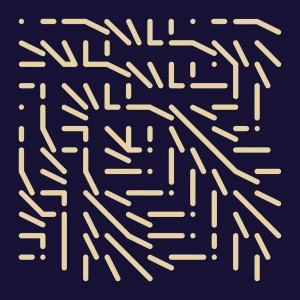# Eight Elements Describing A Square C cont…

Eight Elements Describing A Square  C.  On an expanding grid  (2012)Eight Elements Describing A Square 3x3 CEight Elements Describing A Square 4x4 CEight Elements Describing A Square 5x5 CEight Elements Describing A Square 6x6 CEight Elements Describing A Square 7x7 CEight Elements Describing A Square 8x8 CEight Elements Describing A Square 9x9 CEight Elements Describing A Square 10x10 CEight Elements Describing A Square 11x11 CEight Elements Describing A Square 12x12 C